# Test: Problems On Definite Integrals

## 10 Questions MCQ Test Mathematics (Maths) Class 12 | Test: Problems On Definite Integrals

Description
This mock test of Test: Problems On Definite Integrals for JEE helps you for every JEE entrance exam. This contains 10 Multiple Choice Questions for JEE Test: Problems On Definite Integrals (mcq) to study with solutions a complete question bank. The solved questions answers in this Test: Problems On Definite Integrals quiz give you a good mix of easy questions and tough questions. JEE students definitely take this Test: Problems On Definite Integrals exercise for a better result in the exam. You can find other Test: Problems On Definite Integrals extra questions, long questions & short questions for JEE on EduRev as well by searching above.
QUESTION: 1

### The value of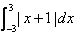is:

Solution:

∫(-3 to 3) (x+1)dx

=  ∫(-3 to -1) (x+1)dx +  ∫(-1 to 3) (x+1) dx

= [x2 + x](-3 to -1) + [x2 + x](-1 to 3)

= [½ - 1 - (9/2 - 3)] + [9/2 + 3 - (½ - 1)]

= -[-4 + 2] + [4 + 4]

= -[-2] + 

= 10

QUESTION: 2

###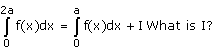Solution:

Option d is correct, because it is the property of definite integral
∫02a f(x) dx = ∫0a f(x) dx + ∫0a f(2a – x) dx

QUESTION: 3

###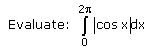Solution:

∫02π|cos x|
[sin x]02π
[sin0 - sin2π]
0 - 0 = 0

QUESTION: 4

Find the value of the integral.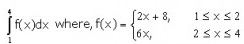Solution:
QUESTION: 5

The value of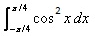is:

Solution:

I = ∫(1+cos2x)/2 dx
⇒ I = 1/2∫(1+cos2x)dx
⇒ I = 1/2∫1dx + 1/2∫cos2xdx
Using the integral formula ∫coskxdx = sinkx/k + c, we have
∫cos2 xdx = 1/2x + 1/2(sin2x)/2 + c
⇒ [1/2x + 1/4sin2x](-π/4 to π/4)
=> [-π/8 - π/8] + [1/4sin(-π/2) - 1/4sin(π/2)]
= - 2π/8 + [-¼ - ¼]
= -π/4 -½
= -(π/4 +½)

QUESTION: 6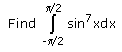Solution:
QUESTION: 7

The value of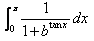is:

Solution:
QUESTION: 8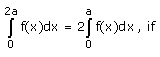Solution:
QUESTION: 9

If f(2a – x) = f(x), then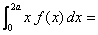Solution:
QUESTION: 10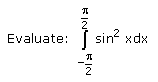Solution:

For sin2(X), we will use the cos double angle formula:
cos(2X) = 1 - 2sin2(X)
The above formula can be rearranged to make sin2(X) the subject:
sin2(X) = 1/2(1 - cos(2X))
You can now rewrite the integration:
∫sin2(X)dX = ∫1/2(1 - cos(2X))dX
Because 1/2 is a constant, we can remove it from the integration to make the calculation simpler. We are now integrating:
1/2 x ∫(1 - cos(2X)) dX
= 1/2 x (X - 1/2sin(2X)) + C]-pi/4 to pi/4
∫sin2(X) dX = [1/2X - 1/4sin(2X)]-pi/4 to pi/4 + C
½[-pi/2] - 1/4sin(2(-pi/4)] - ½[pi/2] - 1/4sin(2(pi/4)]
= π/2